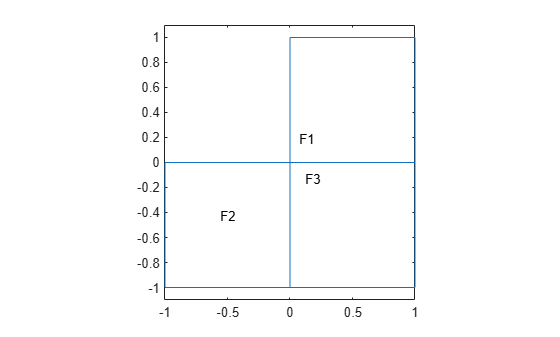# findInitialConditions

Locate active initial conditions

## Syntax

``ic = findInitialConditions(ics,RegionType,RegionID)``

## Description

example

````ic = findInitialConditions(ics,RegionType,RegionID)` returns the active initial condition assignment `ic` for the initial conditions in the specified region.```

## Examples

collapse all

This example shows find the active initial conditions for a region.

Create a PDE model that has a few subdomains.

```model = createpde(); geometryFromEdges(model,@lshapeg); pdegplot(model,"FaceLabels","on") ylim([-1.1,1.1]) axis equal```Set initial conditions on each pair of regions.

```setInitialConditions(model,12,"Face",[1,2]); setInitialConditions(model,13,"Face",[1,3]); setInitialConditions(model,23,"Face",[2,3]);```

Check the initial conditions specification for region 1.

```ics = model.InitialConditions; ic = findInitialConditions(ics,"Face",1)```
```ic = GeometricInitialConditions with properties: RegionType: 'face' RegionID: [1 3] InitialValue: 13 InitialDerivative: [] ```

## Input Arguments

collapse all

Model initial conditions, specified as the `InitialConditions` property of a PDE model. Initial conditions can be complex numbers.

Example: `model.InitialConditions`

Geometric region type, specified as `"Edge"` for a 2-D model, `"Face"` for a 2-D model or 3-D model, or `"Cell"` for a 3-D model.

Example: ```ca = findInitialConditions(ics,"Face",[1,3])```

Data Types: `char` | `string`

Region ID, specified as a vector of positive integers. View the subdomain labels for a 2-D model using `pdegplot(model,"FaceLabels","on")`. Currently, there are no subdomains for 3-D models, so the only acceptable value for a 3-D model is `1`.

Example: ```ca = findInitialConditions(ics,"Face",[1,3])```

Data Types: `double`

## Output Arguments

collapse all

Initial condition assignment, returned as a GeometricInitialConditions Properties or NodalInitialConditions Properties object.

## Version History

Introduced in R2016a